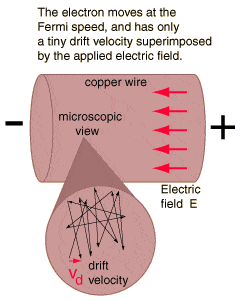# Electron Drift Velocity problem

• himanshushanka
The electron drift velocity is related to the electric field. The Fermian energy is responsible for the electron's velocity.f

#### himanshushanka

Following are the text from an Electrical Engineering Textbook.

"it is seen that contrary to the common but mistaken view,

1. the electron drift velocity is rather very slow
2. is independent of the current flowing
3. independent of the area of the conductor"

first point can be explained by following example,

Assuming a normal current density J= 1.55 x 10^6 A/m^2, n=10^29 for copper conductor and e= 1.6 x 10^-19 coulomb, current density is given by,

J= n.e.v ampere/meter^2, where n is electron density per m^3, e is electron charge and v is drift velocity
we get v=.58 cm/min

However regarding point 2 and 3 above, I am not able to come to any concrete logic.

An enlightenment regarding above two point is highly appreciated.

Dear Jim,

I read the article now. it describes the drift velocity is very low.

As you say, logically, point 2 and 3 seems to be incorrect.

Using the basic treatment of the subject, the electron drift velocity is related to the electric field.
$\mathbf{v}_d = \mu \mathbf{E}$

$\mu$, the electron mobility, is roughly constant for low fields, and depends on the material.

We also have that for an Ohmic material current density is related to the electric field.

$\mathbf{J}= \sigma \mathbf{E}$

So we can put these two together and find that the drift velocity clearly does depend on the current [density]. However based on this it's not apparent that the drift velocity depends on the cross sectional area of the conductor (for DC current at least). If it did, the current wouldn't scale linearly with cross sectional area.

Last edited:
Very good !

velocity is proportional to electric field

Folks accustomed to thinking circuits might logically maintain that for a given current ,
electron drift velocity depends on area

if you halve the area , you'll have to double field to keep current the same.

Thank you Mr Ohm and Mr X.

maybe we need to know what pre-conditions the author established in paragraphs leading up to those statements.

I think 2 and 3 are incorrect.

Have you tried a search on 'electron drift velocity? I saw a good page over at hyperphysics...

http://hyperphysics.phy-astr.gsu.edu/hbase/electric/miccur.html

Using the basic treatment of the subject, the electron drift velocity is related to the electric field.
$\mathbf{v}_d = \mu \mathbf{E}$

$\mu$, the electron mobility, is roughly constant for low fields, and depends on the material.

We also have that for an Ohmic material current density is related to the electric field.

$\mathbf{J}= \sigma \mathbf{E}$

So we can put these two together and find that the drift velocity clearly does depend on the current [density]. However based on this it's not apparent that the drift velocity depends on the cross sectional area of the conductor (for DC current at least). If it did, the current wouldn't scale linearly with cross sectional area.

Yep. The hyperphysics link shows this; Microscopic View of Ohm's Law

The Fermi energy is responsible for the electron's velocity.

In metals, the Fermi energy gives us information about the velocities of the electrons which participate in ordinary electrical conduction. The amount of energy which can be given to an electron in such conduction processes is on the order of micro-electron volts (see copper wire example), so only those electrons very close to the Fermi energy can participate.

Thank you Don ! I have wondered for decades where there was a common sense explanation of this.

So there IS a direct analogy with Brownian motion of fluid molecules in a pipe or river:
Individual molecules clatter around with great velocity related to their thermal energy
but the bulk flow velocity is much lower.Your link should help a LOT with the ongoing difficulty between electron flow and (positive or negative) current flow.
I was really lucky to have that concept imprinted clearly by an excellent high school electronics teacher. He pointed out the wire will melt well before drift approaches an inch per second.